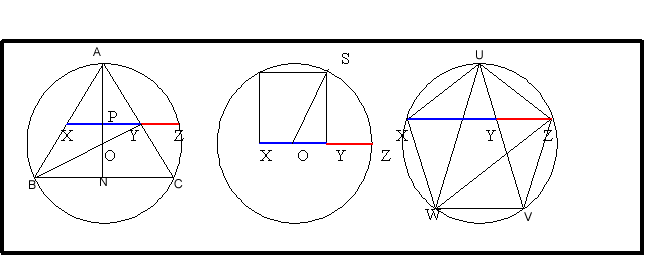#### You may also like### Gold Again

Without using a calculator, computer or tables find the exact values of cos36cos72 and also cos36 - cos72.### Pythagorean Golden Means

Show that the arithmetic mean, geometric mean and harmonic mean of a and b can be the lengths of the sides of a right-angles triangle if and only if a = bx^3, where x is the Golden Ratio.### Golden Triangle

Three triangles ABC, CBD and ABD (where D is a point on AC) are all isosceles. Find all the angles. Prove that the ratio of AB to BC is equal to the golden ratio.

# Gold Yet Again

##### Age 16 to 18Challenge Level

Thank you to Lazarus from Colyton Grammar School and Andrei from Tudor Vianu National College, Bucharest for your solutions to this problem. We see that in all three cases the ratio $XZ$ to $XY$ is the Golden Ratio.In the first diagram, taking the circle to have unit radius, $AO = BO = CO = ZO$.

Triangle $BXO$ is similar to triangle $XPO$ (angles $30^\circ, 60^\circ, 90^\circ$) so $BX = BO\cos30 = {\sqrt 3\over 2}$ and $XO = BO\sin 30 = {1\over 2}$, $OP = XO\sin 30 = {1\over 4}$ and $XP = PY = XO \cos 30 ={\sqrt 3\over 4}$ so $XY = {\sqrt 3 \over 2}$.

Now consider triangle $OPZ$. Angle $OPZ = 90^\circ$. Therefore using Pythagoras' Theorem, $PZ= \sqrt{1 - {1\over 16}}= {\sqrt{15}\over 4}$. So $XZ= XP + PZ = {\sqrt{3}\over 4}\left(1 + \sqrt{5}\right)$ and $${XZ\over XY} = {1\over 2}\left(1 + \sqrt{5}\right)$$ which is the Golden Ratio.

In the second diagram, taking the side of the square $XY$ as $1$ unit and using Pythagoras Theorem for triangle $OSY$ gives $OS={\sqrt 5 \over 2}$ which is the radius of the circle. So $OZ ={\sqrt 5 \over 2}$. Hence $XZ = {1\over 2}\left(1 + \sqrt{5}\right)$ and $${XZ\over XY} = {1\over 2}\left(1 + \sqrt{5}\right)$$ again the Golden Ratio.

In the third diagram take the sides of the pentagon to be $1$ unit long and the chords $XZ$ and $WZ$ etc. to be $x$ units.

Triangles $UXY$, $YVZ$ and $XZW$ are similar isosceles triangles (angles of $36^\circ$, $72^\circ$ and $72^\circ$) with $VY =VZ = XY = 1$ and $YZ = x-1$. We see that the ratio we want to find $XZ/XY = x$.

By similar triangles, taking the ratio of the long to the short sides, we get $${x\over 1 } = {1 \over{x-1}}$$ which gives the quadratic equation $$x^2 - x - 1 = 0$$ with solutions ${1\over 2}\left(1 + \sqrt{5}\right)$ or ${1\over 2}\left(1 - \sqrt{5}\right)$. The latter is negative so does not make sense geometrically so the ratio must be the Golden Ratio: $${1 \over 2}\left(1 + \sqrt{5}\right)$$